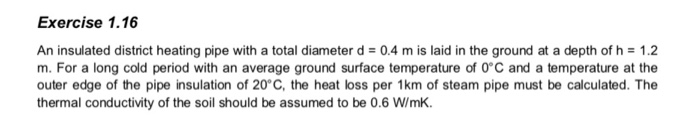# Exercise 1.16 An insulated district heating pipe with a total diameter d = 0.4 m is laid in the ground

###### Question:Exercise 1.16 An insulated district heating pipe with a total diameter d = 0.4 m is laid in the ground at a depth of h = 1.2 m. For a long cold period with an average ground surface temperature of 0°C and a temperature at the outer edge of the pipe insulation of 20°C, the heat loss per 1km of steam pipe must be calculated. The thermal conductivity of the soil should be assumed to be 0.6 W/mk

#### Similar Solved Questions

##### Ms. Thom, age 46, works as an RN in the intensive care unit at a large...
Ms. Thom, age 46, works as an RN in the intensive care unit at a large busy medical center. Over the last three years she has gained 30 lbs and quit attending the fitness classes at the local recreation center. Her co-workers keep trying to get her to come back to fitness class, but she says t...
Is time real?...
##### SAS BASE PROGRAMMING M?C Q PLS HELLP!! Which statement(s) can be used in the GPLOT procedure...
SAS BASE PROGRAMMING M?C Q PLS HELLP!! Which statement(s) can be used in the GPLOT procedure to select observations (records)? Select one: a. WHERE or IF b. None of the other choices c. WHERE O d. IF var r6 -- r11; *Note the two dashes! Select one: O a. Selects the variables that appear in consecut...
##### Displacement
What is the object's displacement between t=1.0s and t=3.0s ifat time t=0s, an object is observed at x=0m; and its position alongthe x-axis follows thisexpression: x=-3t+t^3, where the units for distance and time aremeters and seconds, respectively?...
##### 1. For the cascade amplifier of the figure, calculate the polarization voltages and current colle...
For the cascade amplifier of the figure, calculate the polarization voltages Please clarity answer, thanks!! 1. For the cascade amplifier of the figure, calculate the polarization voltages and current collector of each stage. Also, calculate the voltage gain for each stage and the total voltage gai...
##### Finding solution by graphing solve by graphing x+4y=-6 2x-3y=-1
finding solution by graphing solve by graphing x+4y=-6 2x-3y=-1...